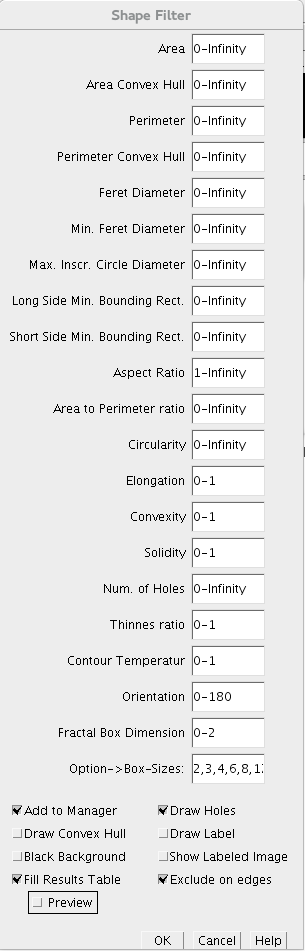# Shape FilterThe content of this page has not been vetted since shifting away from MediaWiki. If you’d like to help, check out the how to help guide!Name Shape Filter Plugin Software ImageJ/Fiji Author Thorsten Wagner Maintainer Thorsten Wagner File shape_filter_x.y.z.jar [1 ] Source Github [2 ] Status active

# General Description

The ImageJ Shape Filter Plugin use the [ij-blob] library to characterize and filter objects in binary scenes by its shape. Therefore, several features are calculated as shown below.If you like to cite the Shape Filter plugin in a scientific publication, please cite:

Wagner, T and Lipinski, H 2013. IJBlob: An ImageJ Library for Connected Component Analysis and Shape Analysis. Journal of Open Research Software 1(1):e6, DOI: http://dx.doi.org/10.5334/jors.ae

## Shape Features

• Area ($$A$$): The area enclosed by the outer contour of an object.
• Area Convex Hull ($$C$$): The area enclosed by the convex hull of the outer contour of an object.
• Perimeter ($$P$$): The perimeter of the outer contour of an object.
• Perimeter Convex Hull ($$H$$): The perimeter of the convex hull of the particle.
• Feret Diameter: The maximum distance between the two parallel tangents touching the particle outline in all directions.
• Min. Feret Diameter: the minimum distance between the two parallel tangents touching the particle outline in all directions.
• Max. Inscr. Circle Diameter: The diameter of the maximum inscribed circle.
• Area eq. circle diameter: Equivalent circle diameter $$2\sqrt{\frac{A}{\pi}}$$
• Long Side Minimum Bounding Rectangle ($$L$$): The larger side of the minimum bounding rectangle.
• Short Side Minimum Bounding Rectangle ($$S$$): The smaller side of the minimum bounding rectangle.
• Aspect Ratio: Defined as $$L/S$$
• Area to Perimeter Ratio: Defined as $$A/P$$
• Circularity: Defined as $$P^{2}/A$$
• Elongation: Defined as $$1 - S/L$$
• Convexity: Defined as $$H/P$$
• Solidity: Defined as $$A/C$$
• Number of Holes: The number of holes inside an object.
• Thinnes Ratio: Inverse proportional to the circularity. Furthermore it normed. It is defined as $$4\pi A/P^{2}$$
• Contour Temperatur: It has a strong relationship to the fractal dimension, defined as $$\left(log_{2}\left(\frac{2P}{P-H}\right)\right)^{-1}$$
• Orientation: The orientation of the major axis from in grad (measured counter clockwise from the positive x axis).
• Fractal Box Dimension: Estimated fractal dimension by the box count algorithm. The default box-sizes are “2,3,4,6,8,12,16,32,64”.

# Installation

You could simply use our update site “Biomedgroup” to install the shape filter plugin.